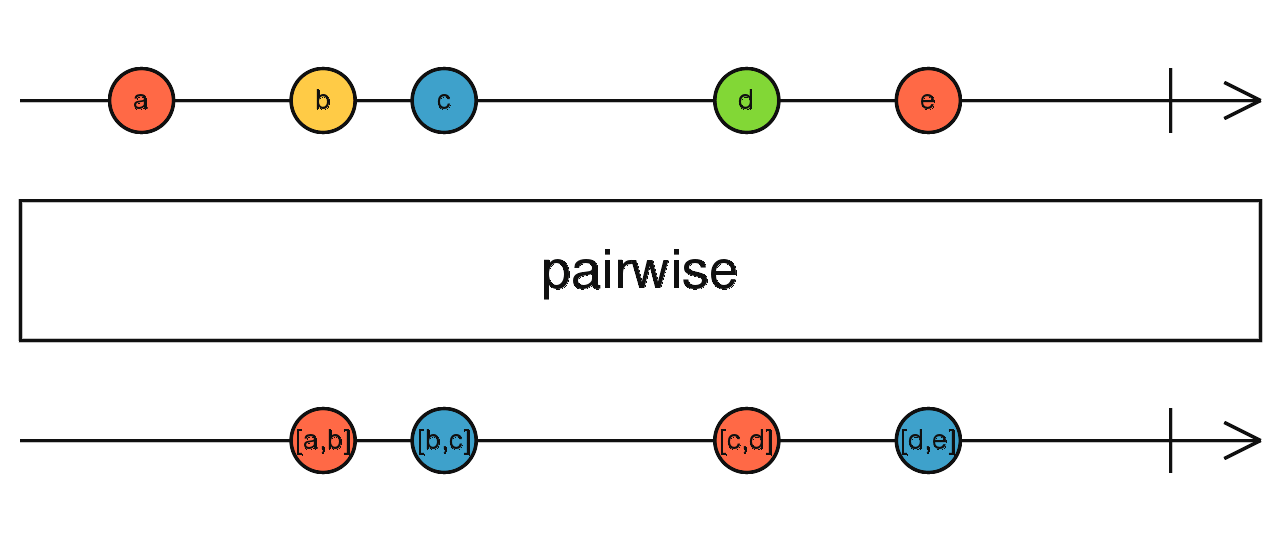/RxJS

# pairwise

`function` `stable`

Groups pairs of consecutive emissions together and emits them as an array of two values.

### `pairwise<T>(): OperatorFunction<T, [T, T]>`

#### Parameters

There are no parameters.

#### Returns

`OperatorFunction<T, [T, T]>`: An Observable of pairs (as arrays) of consecutive values from the source Observable.

## Description

Puts the current value and previous value together as an array, and emits that.The Nth emission from the source Observable will cause the output Observable to emit an array [(N-1)th, Nth] of the previous and the current value, as a pair. For this reason, `pairwise` emits on the second and subsequent emissions from the source Observable, but not on the first emission, because there is no previous value in that case.

## Example

On every click (starting from the second), emit the relative distance to the previous click

```import { fromEvent } from 'rxjs';
import { pairwise, map } from 'rxjs/operators';

const clicks = fromEvent(document, 'click');
const pairs = clicks.pipe(pairwise());
const distance = pairs.pipe(
map(pair => {
const x0 = pair.clientX;
const y0 = pair.clientY;
const x1 = pair.clientX;
const y1 = pair.clientY;
return Math.sqrt(Math.pow(x0 - x1, 2) + Math.pow(y0 - y1, 2));
}),
);
distance.subscribe(x => console.log(x));```